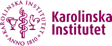# Valentin Vancak

Postdoctoral researcher

Publications

V. Vancak,  A. Sjölander. Sensitivity Analysis of G-estimators to Invalid Instrumental Variables, under review

V. Vancak, Y. Goldberg, S.Z. Levine (2022). The Number Needed to Treat Adjusted for Explanatory Variables and Survival Analysis: Theory and Application, Statistics in Medicine, 41(17), 3299-3320

V. Vancak, Y. Goldberg, S.Z. Levine (2021). Guidelines to Understand the Number Needed to Treat, Evidence-Based Mental Health, 24, 131–136

V. Vancak, Y. Goldberg, S.Z. Levine (2020). Systematic Analysis of the Number Needed to Treat, Statistical Methods in Medical Research, 29, 2393-2410

V. Vancak, Y. Goldberg, S.K. Bar-Lev, B. Boukai (2015). Continuous Statistical Models – With or Without Truncation Parameters?, Mathematical Methods of Statistics, 24, 55-73

## Teaching portfolio

The Hebrew University of Jerusalem, Israel

Dept. of Psychology: R programming for psychology research

University of Haifa, Israel

Dept. of Economics: Introduction to Linear Models

Ph.D. honours program in social sciences: Statistical Modeling with R

Dept. of Evolutionary Biology: Statistical Modeling with R

Teaching Assistant

ORT Braude College of Engineering, Israel

Dept. of Industrial Engineering & Management:  Statistics and Probability, Stochastic Models in Operation Research

Dept. of Mathematics:  Linear Algebra, Univariate Calculus, Multivariate Calculus, Advanced Multivariate Calculus, Advanced Linear Algebra

University of Haifa, Israel

Dept. of Economics: Introduction to Probability, Introduction to Statistics, Introduction to Linear Models

Dept. of Statistics:  Statistics, Probability, Stochastic Processes (M.Sc.), Statistical Inference, Statistical Models I, Statistical Models II, Generalized Linear Models (M.Sc.), Advanced Probability, High Dimensional Data Analysis (M.Sc.), Multivariate Statistics, Applied Statistics I, SAS programming

Faculty of Management (MBA): Quantitative Methods

Dept. of Multidisciplinary Studies: Introduction to Statistics